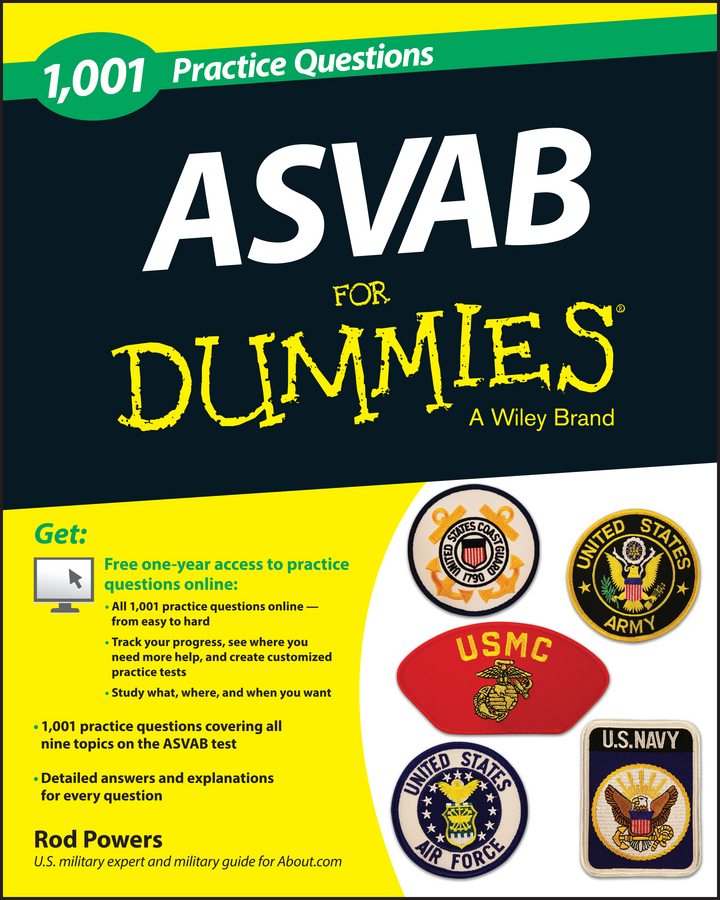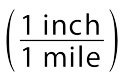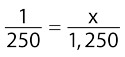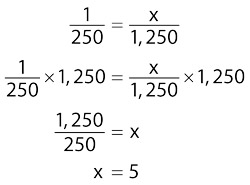##### 1,001 ASVAB Practice Questions For Dummies (+ Free Online Practice)You will need to know how to work with ratios for the Arithmetic Reasoning subtest of the ASVAB. A ratio shows a relationship between two things. For example, if Margaret invested in her tattoo parlor at a 2:1 (or 2 to 1) ratio to her business partner Julie, then Margaret put in \$2 for every \$1 that Julie put in. You can express a ratio as a fraction, so 2:1 is the same as 2/1.

Or suppose you fill up your brand-new, shiny SUV, and you want to compute your gas mileage — miles per gallon. You drive for 240 miles and then refill the tank with 15 gallons of gas, so the ratio of miles to gallons is 240:15. You can compute your gas mileage by dividing the number of miles by the number of gallons: 240 miles ÷15 gallons. You’re getting 16 miles per gallon. Time for a tune up!

## Navigating scale drawings

Scale, particularly when used on the ASVAB, relates to scale drawings. For example, a map drawn to scale may have a 1-inch drawing of a road that represents 1 mile of physical road in the real world. The Arithmetic Reasoning portion of the ASVAB often asks you to calculate a problem based on scale, which can be represented as a standard ratio (1 inch:1 mile) or a fractionOn a map with a scale of 1 inch to 1 mile, the ratio of the scale is represented as 1:1. But questions are never this easy on the ASVAB. You’re more likely to see something like, “If a map has a scale of 1 inch to every 4 miles. . . .” That scale is expressed as the ratio 1:4, or 1/4.

Try your hand with the following common scale problem:

If the scale on a road map is 1 inch = 240 miles, how many inches would represent 1,250 miles?

The problem wants you to determine how many inches on the map represent 1,250 miles if 1 inch is equal to 250 miles. You know that 1 inch = 240 miles, and you also know that some unknown number of inches, which you can call x, equals 1,250 miles. The problem can be expressed as two ratios set equal to each other, known as a proportion:Now all you have to do is solve for x:So if 1 inch is equal to 250 miles, then 5 inches would be equal to 1,250 miles.

Almost every military job makes use of scales, which is why scale-related questions are so common on the ASVAB. Whether you’re reading maps at Mountain Warfare School or organizing trash pickup around the base, you need to use and interpret scales frequently.

## Remembering important rates

A rate is a fixed quantity — a 5% interest rate, for example. It can mean the speed at which one works (John reads at the rate of one page per minute). Or it can mean an amount of money paid based on another amount (life insurance may be purchased at a rate of \$1 per \$100 of coverage). A rate is often a speed, something per a unit of time.

Word problems often ask you to solve problems that involve speed or simple interest rates. Here are two rate formulas you should commit to memory:

• Simple interest: I= Prt, where I represents the amount of interest, P is the principal (the initial amount invested), r is the interest rate, and t is the length of time the money is invested.

• Distance: d = rt, where d represents the distance traveled, r is the rate (speed) of travel, and t is the amount of time traveled.

In a rate, you can generally think of the word per as a division sign. For instance, suppose someone drives 141 miles in 3 hours, and you have to find the average speed. You want the rate of speed in miles per hour, so you take miles (distance) divided by hours (time): 141 miles ÷ 3 hours = 47 miles/hour.

Using algebra, you can rearrange the distance formula to say the same thing: d ÷ t = r.